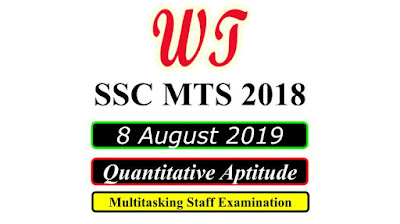Type Here to Get Search Results !

# [Download] SSC MTS 8 August 2019, Quantitative Questions PDF

Download SSC (Staff Selection Commission) MTS - Multi Tasking (Non-Technical) Staff Examination 2018 Tier 1 All Quantitative Aptitude Questions , Was Held on 8 August 2019 All Quantitative Questions PDF With Answers.

Table of Content

Table of Content (toc)

## SSC MTS 8 August 2019 All Shifts Quantitative Questions PDF Download FreeSSC MTS 8 August 2019 All Shifts Quantitative Questions PDF Download Free

### SSC MTS 8 August 2019 Shift 1 Quantitative Questions

Instructions
For the following questions answer them individually
Q1:-The difference in selling prices of an article when sold at 15% profit and 17% loss is ₹96.If it is sold at 10% profit, then what is the selling price?
a.    ₹345
b.    ₹360
c.    ₹315
d.    ₹330
Q3:-A sum of ₹2000 is invested on simple interest for three years at the rate of 10% per annum, then the amount will be:
a.    ₹2900
b.    ₹2600
c.    ₹2300
d.    ₹2500
Q4:-The proportion of the number of students in three classes is 1 : 2 : 3. If 20 students are included in each class, then the proportion becomes 3 : 5 : 7. What was initially the total number of students in the three classes?
a.    200
b.    280
c.    220
d.    240
Q5:-Marked price of an article is ₹2600, which is 30% more than the cost price. If the profit is 45%, then what will be the selling price of the article?
a.    ₹3100
b.    ₹3900
c.    ₹2700
d.    ₹2900
Q6:-A train with 72 km/h speed crosses a stationary pole in 35 seconds. How much time (in minutes) does it take to cross a 1.1 km long bridge?
a.    2.5
b.    3
c.    2
d.    1.5
Q7:-The average weight of 18 boys in a group is 35 kg. If four new boys of weights 20 kg, 22 kg 26 kg and 28 kg are added to the group, then what is the average weight of the newly formed group?
a.    34 years
b.    32 years
c.    35 years
d.    33 years

### SSC MTS 8 August 2019 Shift 2 Quantitative Questions

Instructions
For the following questions answer them individually
Q1:-Three successive discounts of 20%, 20% and 30% are offered on an article. If the marked price of the article is ₹750, then what will be selling price?
a.    ₹326
b.    ₹375
c.    ₹348
d.    ₹336
Q3:-The sum of the present ages of a father and his son is 78 years. After five years, the ratio of their ages becomes 7 : 4. What is the present age (in years) of the father?
a.    45
b.    51
c.    56
d.    50
Q4:- Three positive numbers are in the proportion 3 : 4 : 6. If the sum of their squares is 244, then what is the largest number?
a.    16
b.    8
c.    12
d.    6
Q7:-A man travels from P to Q at the speed of 60 km/h and travels from Q to P at the speed of 90 km/h. What is the average speed of the man for the whole journey?
a.    75 km/h
b.    78 km/h
c.    70 km/h
d.    72 km/h
Q8:-A bag contains one rupee, 50 paise and 25 paise coins in the proportion 5 : 7 : 9. If the total amount in the bag is ₹430, then how many 25 paise coins are there?
a.    400
b.    360
c.    380
d.    340

### SSC MTS 8 August 2019 Shift 3 Quantitative Questions

Instructions
For the following questions answer them individually
Q1:-What is the average of first 15 odd numbers among the natural numbers?
a.    18
b.    15
c.    16
d.    17
Q2:-What is the circumference of the largest circle which can be inscribed in a square of side 14 cm?(Take )
a.    66 m
b.    88 cm
c.    22 cm
d.    44 cm
Q3:-The marked price of an article is ₹3040. If the discount offered on this article is 20%, then what will be the selling price?
a.    ₹2412
b.    ₹3262
c.    ₹2432
d.    ₹3132
Q4:-The present age of a Manoj is twice the sum of the ages of his two children. After 20 years, the age of Manoj will become equal to the sum of the ages of his two children. What is the present age of Manoj?
a.    40 years
b.    30 years
c.    36 years
d.    35 years
Q5:-A train starts from A at 6 AM and reaches B at 11 AM on the same day. Another train starts from B at 8 AM and reaches A at 3 PM on the same day. At what time the two trains will have crossed each other?
a.    9:45 AM
b.    8:45 AM
c.    10:30 AM
d.    7:45 AM

SSC MTS Previous Year Paper

SSC MTS 2018

SSC MTS Reasoning Questions

SSC MTS Quantitative Questions

SSC MTS General English Questions

SSC MTS General Knowledge